International
Tables for
Crystallography
Volume F
Crystallography of biological macromolecules
Edited by M. G. Rossmann and E. Arnold

International Tables for Crystallography (2006). Vol. F, ch. 25.2, pp. 711-712   | 1 | 2 |

## Section 25.2.3.2. The CNS language

A. T. Brunger,v* P. D. Adams,e W. L. DeLano,f P. Gros,g R. W. Grosse-Kunstleve,e J.-S. Jiang,h N. S. Pannu,i R. J. Read,j L. M. Ricek and T. Simonsonl

#### 25.2.3.2. The CNS language

| top | pdf |

One of the key features of the CNS language is symbolic data structure manipulation, for example,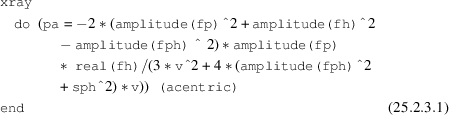which is equivalent to the following mathematical expression for all acentric indices h,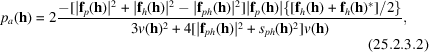where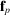[fp' in equation (25.2.3.1)] is the native' structure-factor array,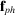[fph' in equation (25.2.3.1)] is the derivative structure-factor array,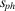[sph' in equation (25.2.3.1)] is the corresponding experimental σ, v is the expectation value for the lack of closure (including lack of isomorphism and errors in the heavy-atom model), and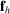[fh' in equation (25.2.3.1)] is the calculated heavy-atom structure-factor array. This expression computes the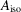coefficient of the phase probability distribution for single isomorphous replacement described by Hendrickson & Lattman (1970)and Blundell & Johnson (1976).

The expression in equation (25.2.3.1)is computed for the specified subset of reflections (acentric)'. This expression means that only the selected (in this case all acentric) reflections are used. More sophisticated selections are possible, e.g.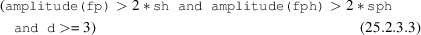selects all reflections with Bragg spacing, d, greater than 3 Å for which both native (fp) and derivative (fph) amplitudes are greater than two times their corresponding σ values (sh' and sph', respectively). Extensive use of this structure-factor selection facility is made for cross-validating statistical properties, such as R values (Brünger, 1992b),values (Kleywegt & Brünger, 1996; Read, 1997) and maximum-likelihood functions (Pannu & Read, 1996a; Adams et al., 1997).

Similar operations exist for electron-density maps, e.g.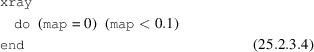is an example of a truncation operation: all map values less than 0.1 are set to 0. Atoms can be selected based on a number of atomic properties and descriptors, e.g.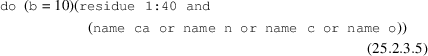sets the B factors of all polypeptide backbone atoms of residues 1 through 40 to 10 Å2.

Operations exist between data structures, e.g. real- and reciprocal-space arrays, and atom properties. For example, Fourier transformations between real and reciprocal space can be accomplished by the following CNS commands: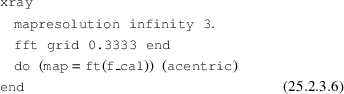which computes a map on a 1 Å grid by Fourier transformation of the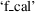array for all acentric reflections.

Atoms can be associated with calculated structure factors, e.g.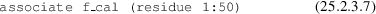This statement will associate the reciprocal-space array f_cal' with the atoms belonging to residues 1 through 50. These structure-factor associations are used in the symbolic target functions described below.

There are no predefined reciprocal- or real-space arrays in CNS. Dynamic memory allocation allows one to carry out operations on arbitrarily large data sets with many individual entries (e.g. derivative diffraction data) without the need to recompile the source code. The various reciprocal-space structure-factor arrays must therefore be declared and their type specified prior to invoking them. For example, a reciprocal-space array with real values, such as observed amplitudes, is declared by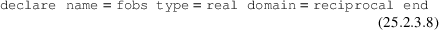Reciprocal-space arrays can be grouped. For example, Hendrickson & Lattman (1970)coefficients are represented as a group of four reciprocal-space structure-factor arrays,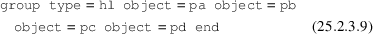where pa', pb', pc' and `pd' refer to the individual arrays. This group statement indicates to CNS that the specified arrays need to be transformed together when reflection indices are changed, e.g. during expansion of the diffraction data to space group P1.

### References

Adams, P. D., Pannu, N. S., Read, R. J. & Brünger, A. T. (1997). Cross-validated maximum likelihood enhances crystallographic simulated annealing refinement. Proc. Natl Acad. Sci. USA, 94, 5018–5023.Google Scholar
Blundell, T. L. & Johnson, L. N. (1976). Protein crystallography, pp. 375–377. London: Academic Press.Google Scholar
Brünger, A. T. (1992b). Free R value: a novel statistical quantity for assessing the accuracy of crystal structures. Nature (London), 355, 472–475.Google Scholar
Hendrickson, W. A. & Lattman, E. E. (1970). Representation of phase probability distributions for simplified combination of independent phase information. Acta Cryst. B26, 136–143.Google Scholar
Kleywegt, G. J. & Brünger, A. T. (1996). Checking your imagination: applications of the free R value. Structure, 4, 897–904.Google Scholar
Pannu, N. S. & Read, R. J. (1996a). Improved structure refinement through maximum likelihood. Acta Cryst. A52, 659–668.Google Scholar
Read, R. J. (1997). Model phases: probabilities and bias. Methods Enzymol. 277, 110–128.Google Scholar